### Home > CAAC > Chapter 7 > Lesson 7.3.1 > Problem7-90

7-90.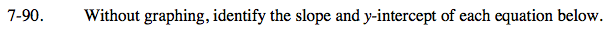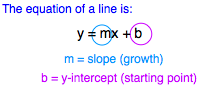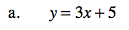See the hint above.

Slope = 3
y-intercept = (0,5)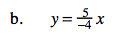This equation is the same as:

$y=\frac{5}{-4}x+0.$

$\text{Slope }=\frac{5}{-4}x$

y-intercept = (0,0)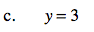If there is no x-value in the equation, can there be a slope or is it 0?

Slope = 0
y-intercept = (0,3)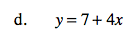See part (a) and the first hint in the problem.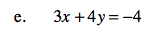Solve the equation for y. By doing this, you put the equation in y = mx + b form.

Subtract by 3x on each side.

4y = −3x − 4

Divide by 4 on both sides.

$y=\frac{-3}{4}x-1$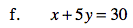See part (e) and the first hint in the problem.